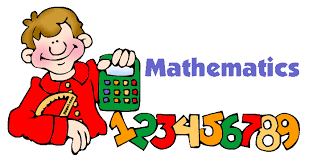Writing Inequalities
3 years ago
kharalambopoulos
Save
Edit
Host a game
Live GameLive
Homework
Solo Practice
Practice14 QuestionsShow answers
• Question 1
30 seconds
Q. x is less than or equal to 24
x > 24
x < 24
x ≤ 24
x ≥ 24
• Question 2
30 seconds
Q. you must be at least 48 inches to ride the roller coaster.
x > 48
x < 48
x ≤ 48
x ≥ 48
• Question 3
30 seconds
Q. You can spend no more than 20 dollars on your mom's birthday present.
x > 20
x < 20
x ≤ 20
x ≥ 20
• Question 4
30 seconds
Q. You and your friends ate more than 8 slices of pizza at the party.
x > 8
x < 8
x ≤ 8
x ≥ 8
• Question 5
30 seconds
Q. You spent less than 5 hours on homework each night.
x > 5
x < 5
x ≤ 5
x ≥ 5
• Question 6
60 seconds
Q. Edith must read for a minimum of 20 minutes every night.
x > 20
x < 20
x ≥ 20
x ≤ 20
• Question 7
30 seconds
Q. All students must get at least a 60% for the year in order to pass math.
x = 60
x > 60
x ≥ 60
x ≤ 60
• Question 8
30 secondsQ. What inequality does the number line graph represent
x ≤ 4
x ≥ -4
x < -4
x < 4
• Question 9
30 secondsQ. What inequality does the number line graph represent?
x ≥ 3
x > 3
x < -3
x ≤ -3
• Question 10
30 seconds
Q. When graphing b< 6 would you use an open or closed circle?
Open
closed
• Question 11
60 secondsQ. Which inequality is represented by this graph? hint: don't be tricked by the variable on the left
2.75 > d
2.75 < d
2.75 ≥ d
2.75 ≤ d
• Question 12
30 secondsQ. What inequality does the number line graph represent?
x ≥ 3
x > 3
x < -3
x ≤ 3
• Question 13
30 seconds
Q. When graphing b< 6 would you use an open or closed circle?